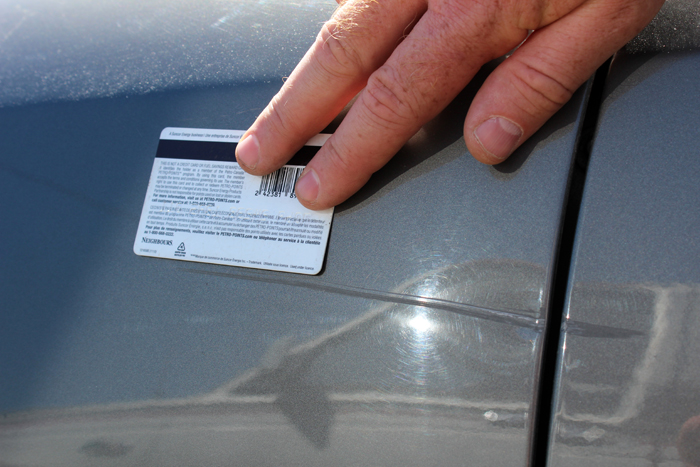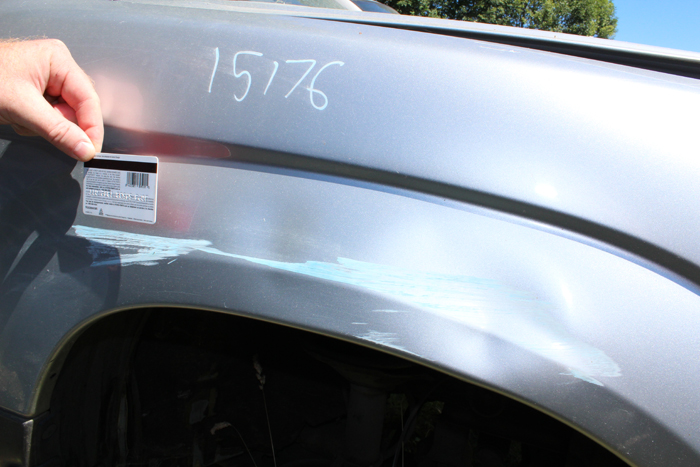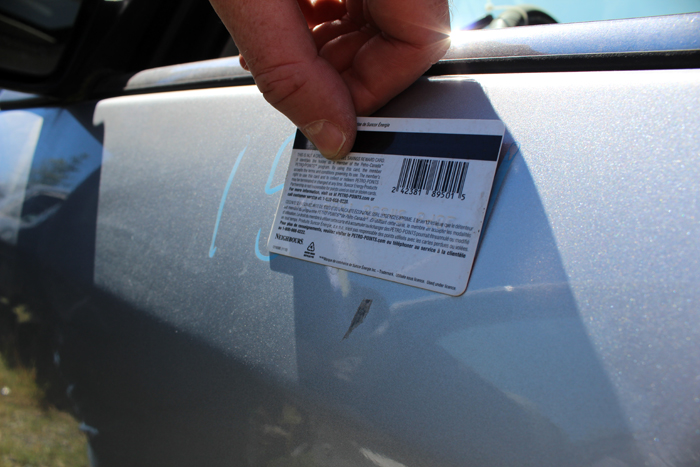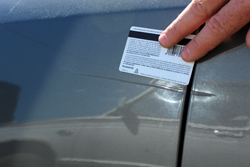# Module 2, Lesson 4 Copy

## Preamble

Approximate time to complete this lesson: 30 minutes.

## Lesson 4 Video

To begin Lesson 4, watch this video (5 minutes, 21 seconds).## Review of Lesson 4

### Damage Type

The second character in the code is the type of damage and is indicated by a letter.

B=Burn
C=Crease
R=Rust
D=Dent
E=Bent
H=Hail
J=Crack
K=Buckle
L=Lip
P=Parking Lot Damage
S=Scratch
T=Paint Problem

Here are examples of each of these Damage Types:

### Units of Damage

The third character defines the amount of damage there is to a part. A whole number is used to express the amount of damage and is referred to as a ‘Unit’.

A ‘Unit’ is defined as: “Damage not exceeding the surface area of a standard sized credit card”.

A unit is not an hour, which is a common but subjective description of damage. Hours represent the time needed to repair a part. Recyclers and collision repair shops seldom agree on the time needed to repair damage.

Let’s look at some examples:Here we can see the area is greater than one credit card. So the unit of damage here is 2.Here we can see that the amount of damage covers an area greater than 2 units.

A, B, C, NIQ, & X grade parts are used to denote the quality grade of a part. A grade parts are one unit or less of necessary repair. B grade parts are two units or less of necessary repair.
C grade parts are more than two units of necessary repair.

NIQ or negative information quality grade parts are automatically down-graded to C. NIQ is also sometimes referred to as Non-Insurance Quality.

X graded parts do not contain enough data for the recycler to grade the part.

### Assigning an A, B or C grade to parts

Grading is based on necessary repairs and is expressed as ‘units’. A unit is a number that represents damage that can be covered by a standard sized credit card.

• A grade = one unit or less
• B grade = two units or less
• C grade = more than two units

Body Parts Grading (Sheet Metal Parts) Examples:Here we can see that the unit of damage is One because it can be covered with a credit card. This part would then be graded as an “A” Grade part.The damage of this part can be covered with two standard sized credit cards so we could grade this part as “B” Grade.

In this example we can see that damage is greater than 2 units, so we would grade this part a “C” or “non-insurance quality”.